Blog Post

Reactive Power Management in Power Distribution System

An in-depth discussion on how to manage reactive power in utility network. This is important basic concept to know in the times of increasing EV and solar renewables penetration.

6 mins

Introduction

Most of the loads in a modern power distribution system are inductive in nature. Typical examples being, loaded overhead lines, motors, transformers, cables, drives, and fluorescent lights. These inductive loads consume both active and reactive power. Active power is required to meet real output requirements and inductive reactive power is required to maintain the magnetic field in the core. Phasor diagram of these parameters is shown in Fig 1.1(a) and 1.1(b). The phasor diagram is described below.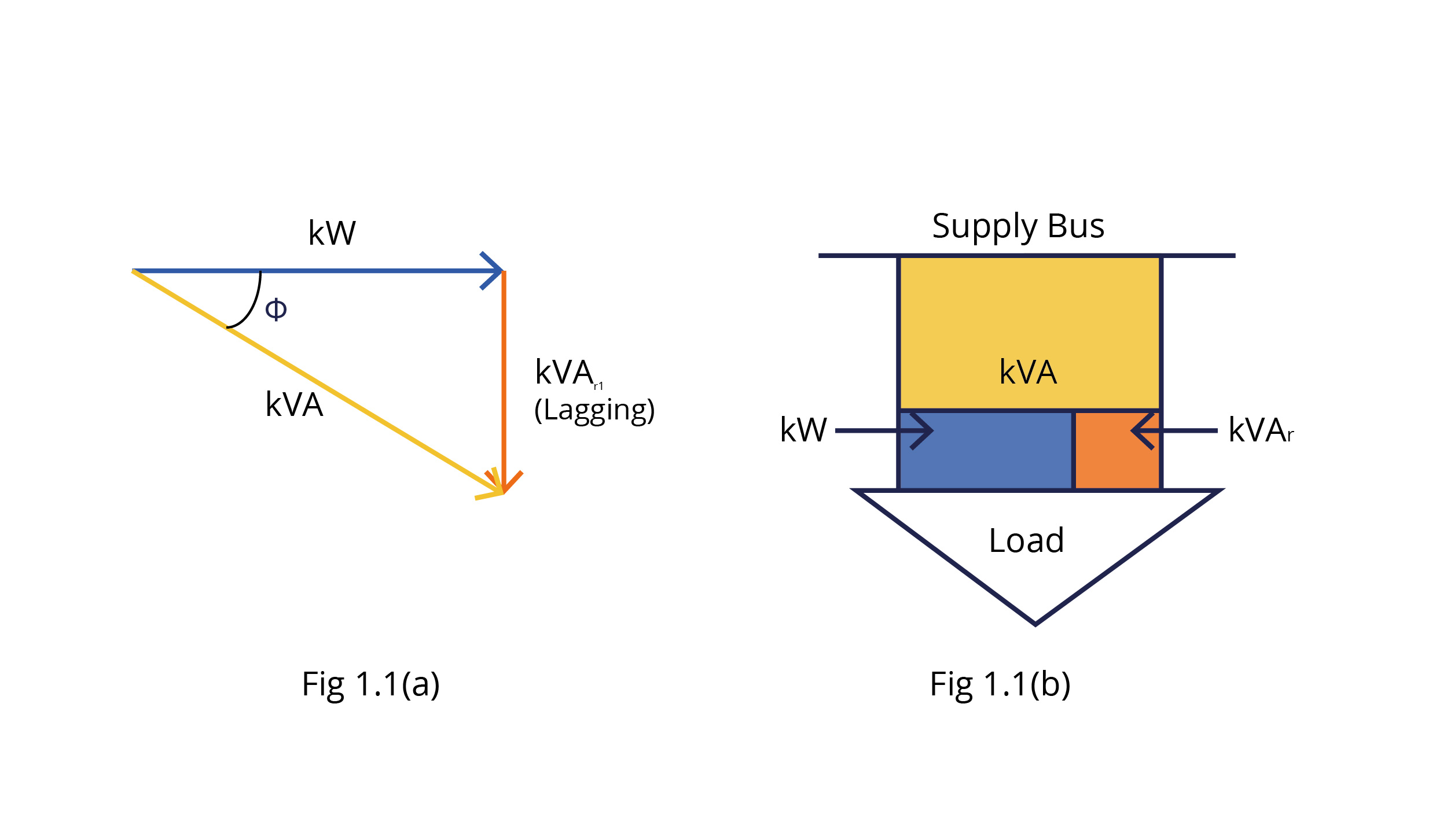Cos Φ = Power factor,

kW = Active power,

kVAri = Reactive power

kVA = Apparent power = (kW2 + kVAri2)1/2

Given this, the power supply system should be capable of supplying both active and inductive reactive power. Presence of inductive reactive power results in the power factor being less than unity and lagging in nature, which in turn results in the following ill effects on a power distribution system.

• Higher current flow from the supply side.

• Reduced efficiency of the power distribution system.

• Higher kVA demand from the supply side.

• Higher electricity bills due to the increase in fixed charges based on kVA demand.

• Levy of penalty by DISCOM if power factor < 0.9 lagging.

Thus, we need to reduce the reactive power to reduce all of the above ill effects. The most effective method for this is increasing the power factor of a power distribution system. The methodology used to achieve a higher power factor under modern application conditions is REACTIVE POWER MANAGEMENT. It can also be said that “REACTIVE POWER MANAGEMENT” is a tool for improvement in the power factor of a distribution system. Hence, both of these are two faces of the same coin.  The methodologies used for power factor improvement are mentioned below

• Installation of fixed type power capacitors

• Installation of Automatic Power Factor Correction System using multiple power capacitors. Automatic Power Factor Correction System uses the technique of automatic switching of capacitor banks based on the requirement of reactive power to be fed by capacitors to maintain power factor at some predetermined value say 0.99.

The concept of power factor improvement is shown in Fig 1.2(a). Here it is shown that kVAR1 is reactive power fed by the supply-side before the installation of capacitors. (kVAR1 - kVAR2) is reactive power fed by the capacitor. kVAR2 is new resultant reactive power to be fed by the supply system after the installation of capacitors. It can be seen that kVAR2 < kVAR1. In Fig 1.2(b) also, it is shown that kVARc is reactive power fed by the capacitors.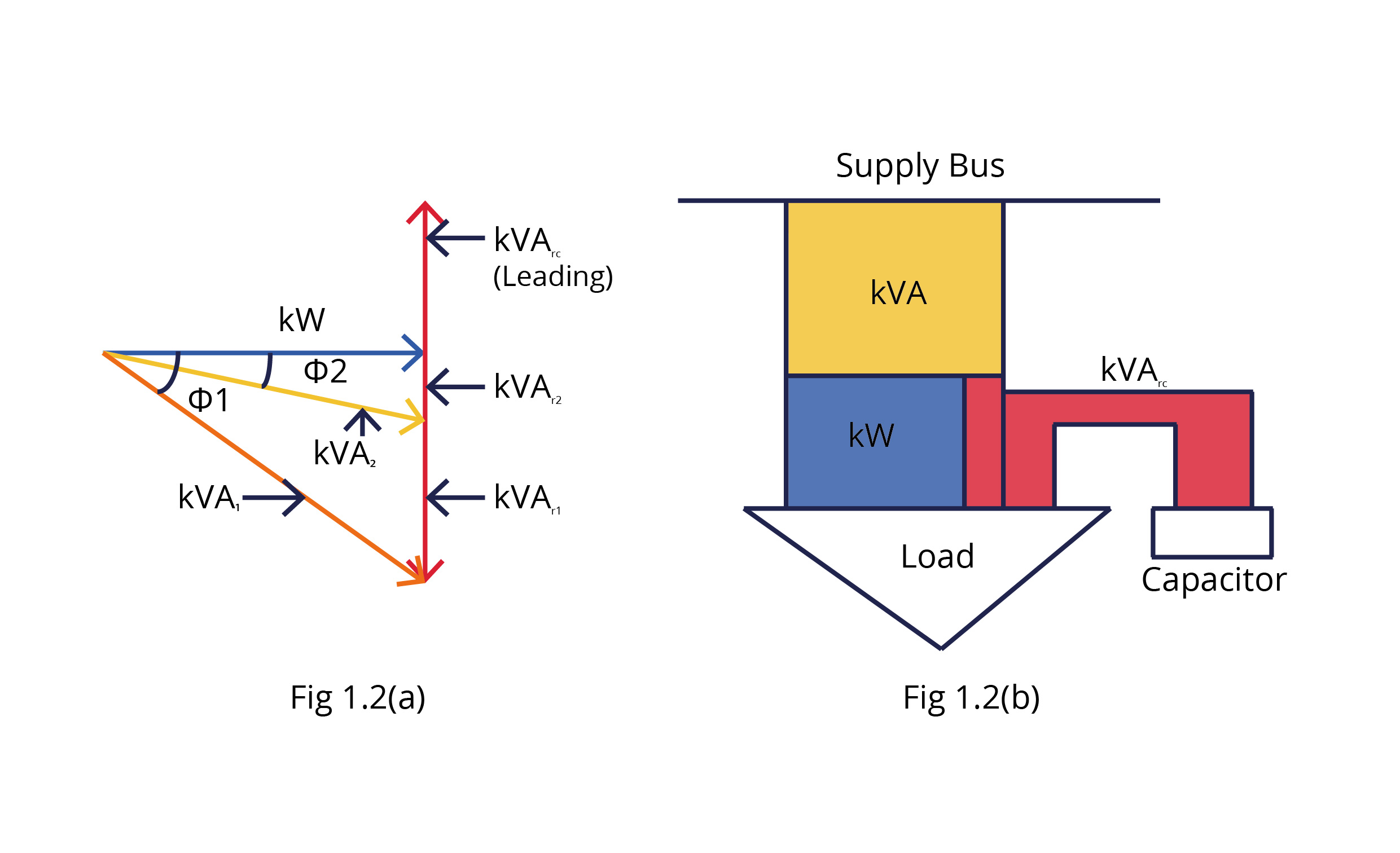Benefits of REACTIVE POWER MANAGEMENT

• Decrease in current flow from the supply side.

• Higher efficiency due to decrease in losses in loaded overhead lines, transformers, and cables.

• Reduction in fixed charges in electricity bill due to lower kVA demand.

• Incentive in electricity bill by 10% of energy charges for every 0.1 increase in power factor above 0.95 lagging.

• Elimination of penalty in electricity bill due to power factor < 0.9 lagging.

• Improved voltage at receiving end side of the power system.

• Increased life of transformers, cables, and switchgear due to lower operating temperatures.

• Due to the reduction in kVA demand, additional loads can be added to the same capacity distribution system.

Fig 2.1(a) shows a simplified distribution system with a power factor of 0.75 without using capacitors. Fig 2.1(b) shows the same distribution system with a power factor of 0.95 by using capacitors.

kVA = kW + kVAR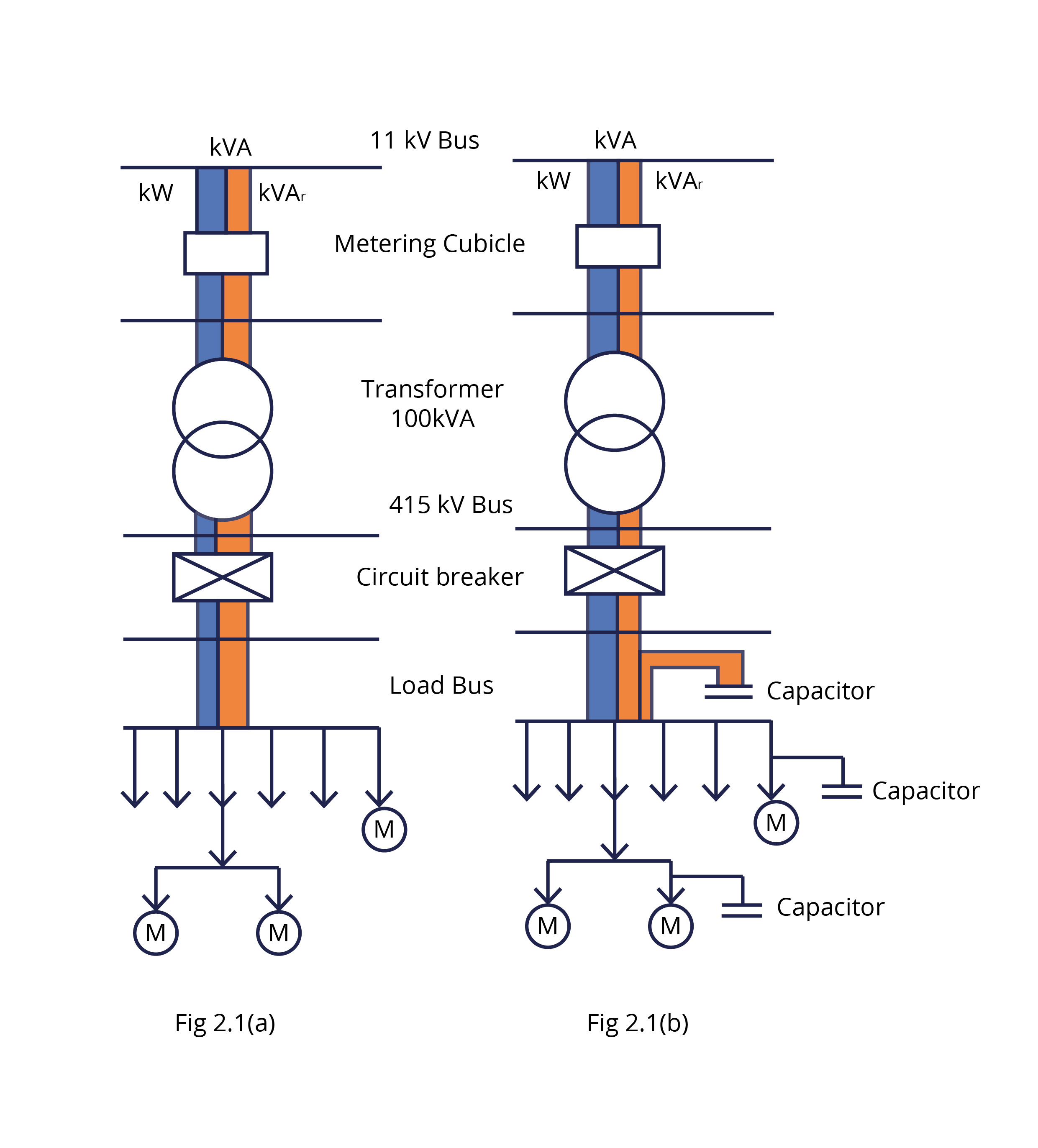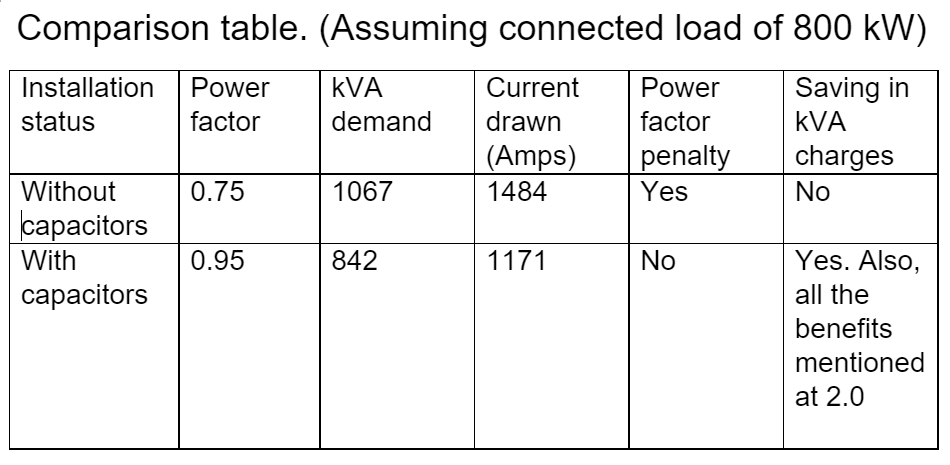Modes of capacitor compensation

3.1 – Providing compensation at the main incomer to power distribution system bus bar is called central compensation. This is shown in Fig. 3.1. Here capacitor is installed at position No. 1.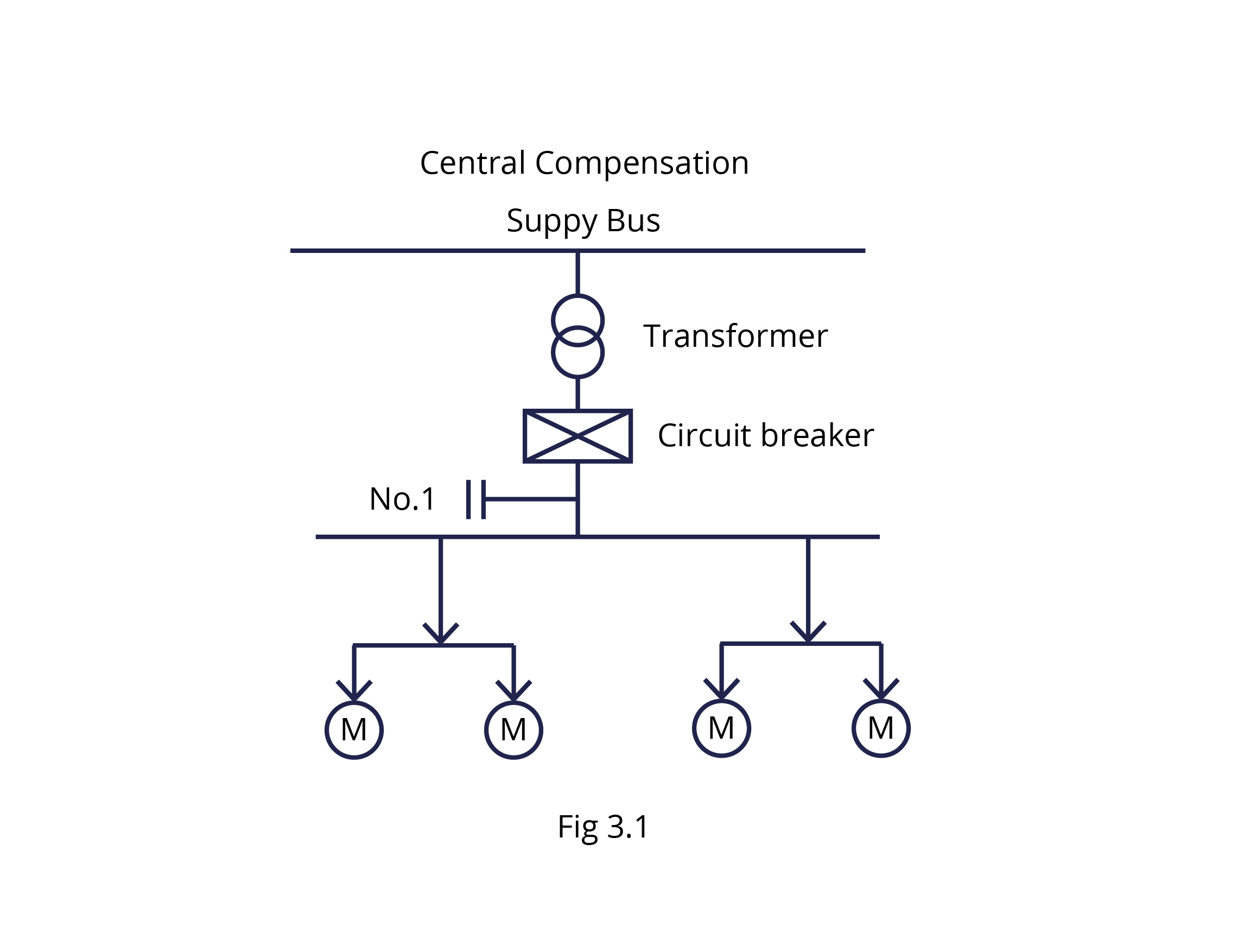3.2 – Providing capacitor compensation at

• Main incomer – Central compensation (position No.1).

• At power distribution boards – Group compensation (position No.2)

This is suitable for installations where there is a number of power distribution boards for various load feeders. This is shown in Fig. 3.2.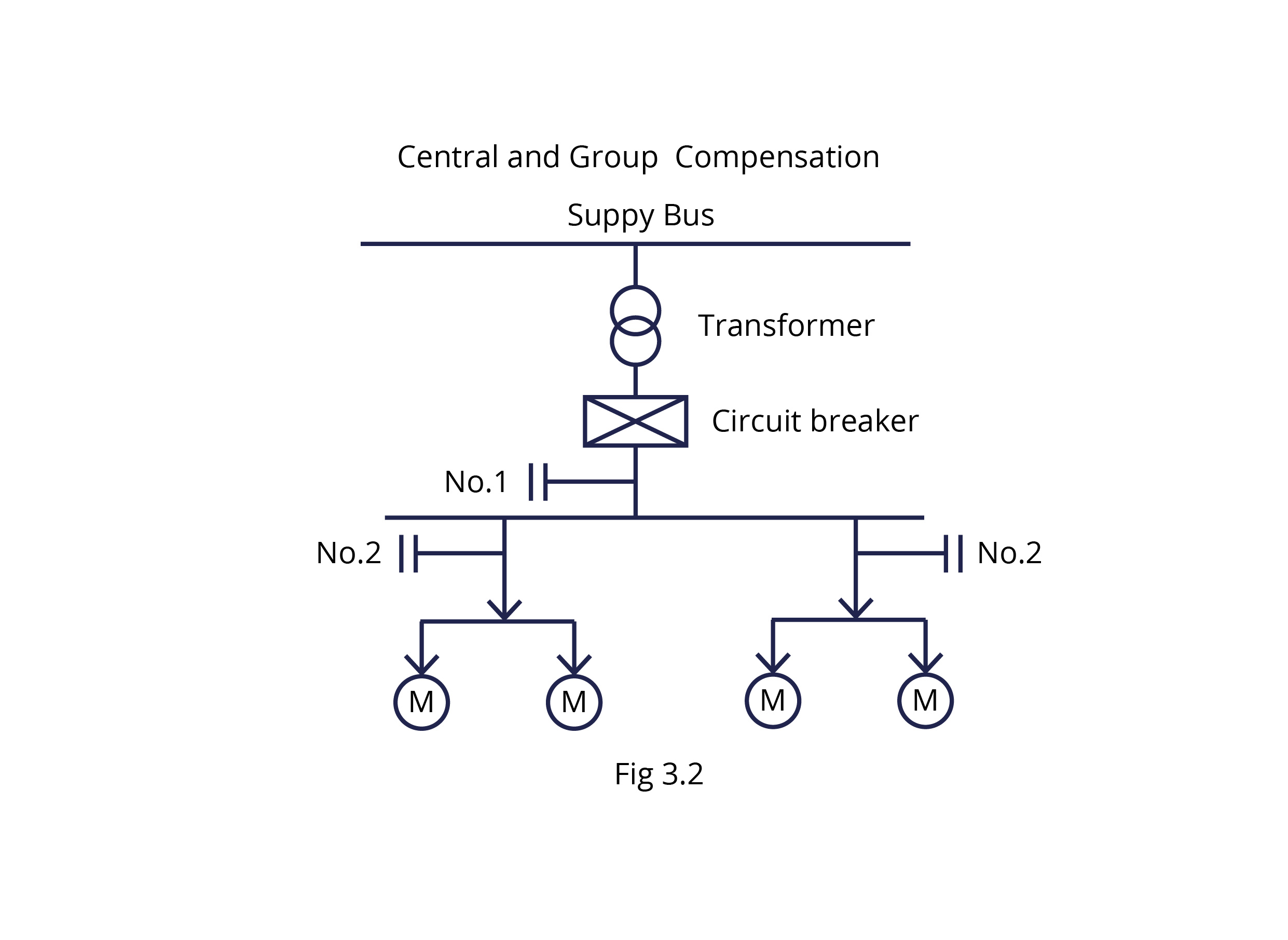3.3 – Providing capacitor compensation at all positions as described below.

• At the main incomer bus – Central compensation (position No.1)

• At Power distribution boards – Group compensation (position No.2)

• At individual load terminals – Individual compensation (position No.3)

This is suitable for installations consisting of the main receiving station, substations, several load feeders, and a variety of loads. This is shown in Fig. 3.3.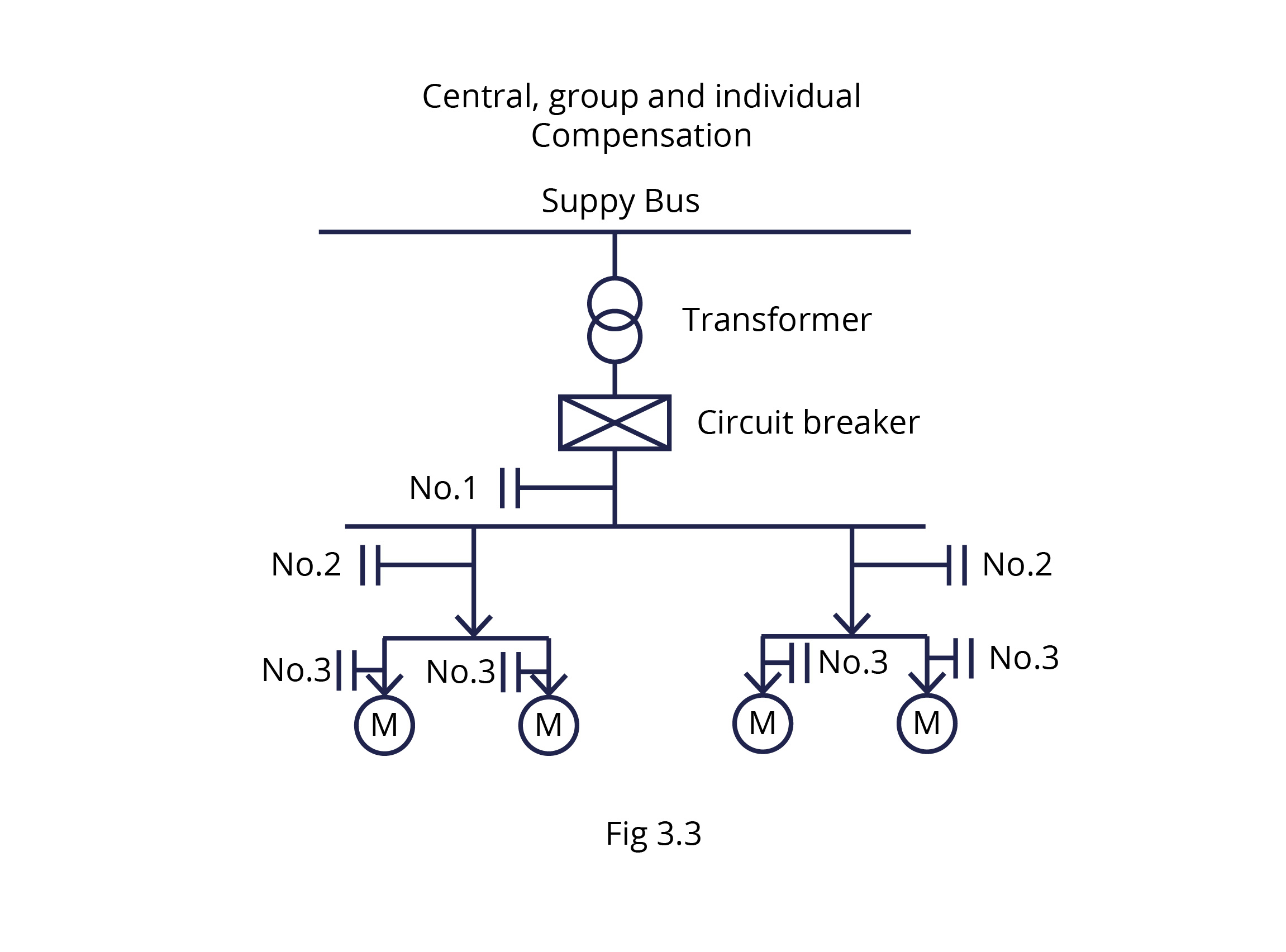Calculation of kVAr required

The estimation of kVAr required for compensation to achieve desired power factor is generally done depending on the type of loads to be compensated. For this purpose, the tables and formulae given in this section may be used.

4.1 – Capacitor kVAr for AC induction motors.

Table 4.1 gives the recommended ratings of power capacitors, which are to be used directly with 3 ph. AC induction motors as at position No. 3 of fig 3.3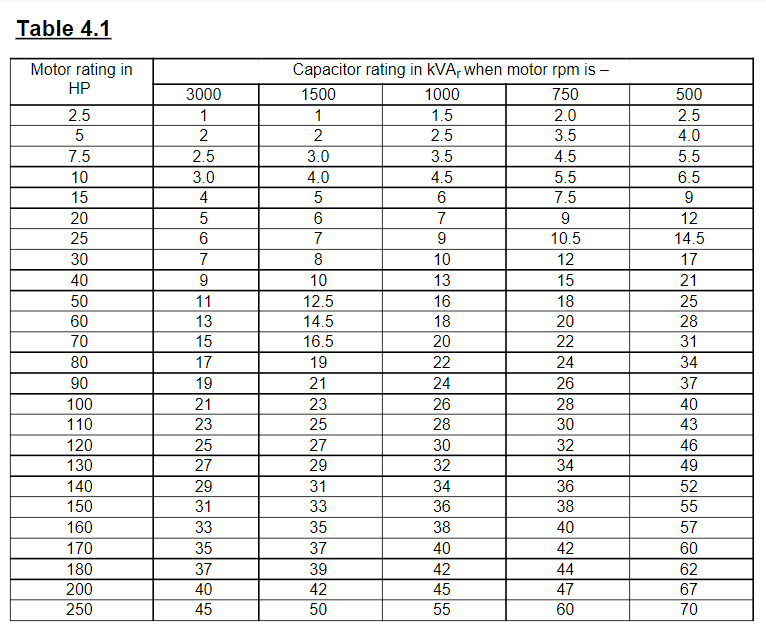Note :

1. It is considered uneconomical to improve power factor (pf) for motors of ratings below 15 hp in power distribution systems.

1. For motor ratings above 250 hp, the capacitor kVAr rating would be about 25% of the motor rating in hp.

1. In all cases, it should be ensured that the capacitor current at rated voltage is always less than 90% of the no-load current of the motor. This is necessary to prevent excessive surge voltages in the event of an interruption in the power supply. This is harmful to both motor and capacitor.

1. The capacitor kVAr values indicated in Table 4.1 are after taking into consideration the condition specified in note 3 and assuming motor loading > 80%.

4.2 Capacitor kVAr for Power distribution systems –

For power distribution systems, the capacitors are to be installed at position No.1 of Fig. 3.1. Initial pf, final pf, and multiplying factors are shown in table 4.2. The operating load kW and its average pf can be obtained from the electricity bill. Else, it can also be calculated from the following formula.

Average pf = kWh/kVAh, operating load kW = kVA demand X average pf. The average pf is considered as initial pf and the final pf is the pf to be achieved by “REACTIVE POWER MANAGEMENT”. This can be calculated using multiplying factor given in table 4.2, This is shown in the example given below.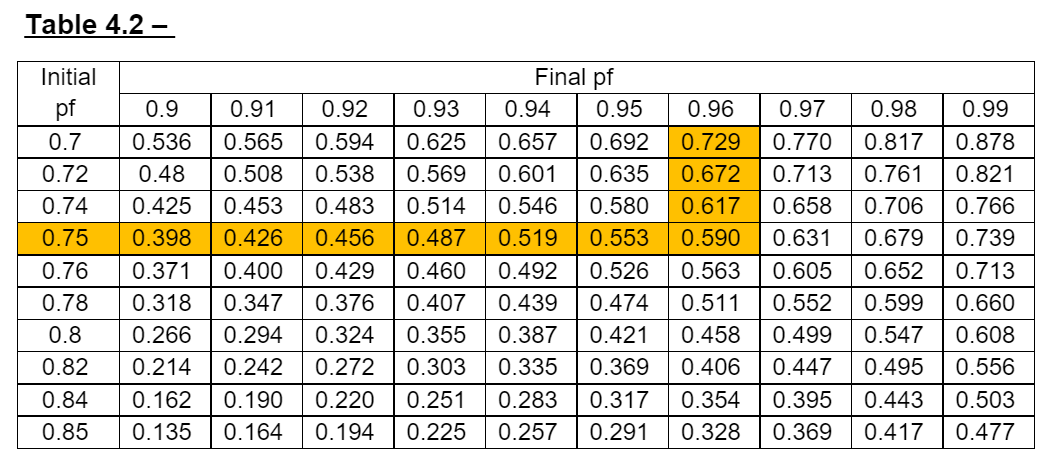Example –

Calculate the required kVAr compensation for a 500 kW installation to improve the pf from 0.75 to 0.96.

kVAr = kW X multiplying factor from table 4.2 = 500 X 0.59 = 295 kVAr

Note –

The table 4.2 is based on following formulae.

Multiplying factor = (tan Φ1 – tan Φ2 )

kVAr required = kW (tan Φ1 – tan Φ2 ), where Φ1 = Cos-1(pf1), Φ2 = Cos-1(pf2)

pf1 and pf2 are initial and final power factors respectively.